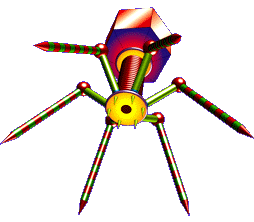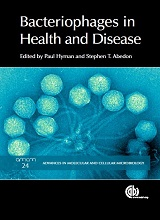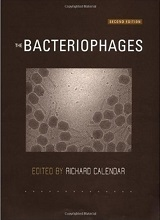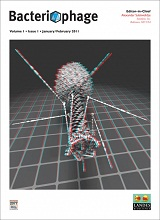# Biology as Poetry: Evolutionary Biology## Hardy-Weinberg Theorem

Sorry about the math, but the italics are exam questions… ☺

For a one locus, two allele, diploid system, of allele frequencies p and q, the following given random mating will hold: p2 + 2pq + q2 = (p + q) × (p + q) = 1.

Other assumptions are that mutation, genetic drift, migration, and natural selection are not occurring. Given these assumptions then p2 and 2pq and q2 are the expected frequencies of three possible genotypes, i.e., PP, PQ, and QQ, here using the upper case P and Q as allele names. For further explanation, see Hardy-Weinberg equilibrium.

For an equivalent three-allele system, the equation instead would consist of:

p2 + q2 + r2 + 2pq + 2qr + 2pr = (p + q + r) × (p + q + r) = 1

To reiterate for the two-allele system:

p2 + 2pq + q2 = 1.

(p + q) × (p + q) = 1.

And, mathematically, this occurs because p + q = 1. That is, since there are only two alleles in the system, in this example, the sum their frequencies will account for all of the alleles in the system, i.e., 100%, which is another way of saying 1.

Furthermore, the odds of generating a specific homozygote – e.g., PP or QQ – are either p2 or q2 (i.e., the odds of picking one allele twice in a row or, instead, the other allele twice in a row). The odds of generating the heterozygote, i.e., PQ, are 2pq, and this actually is pq + qp, i.e., the odds of picking one allele type and then the other plus the odds of picking the same combination of alleles but in the opposite order.

Similarly, for the three-allele system also illustrated above, p + q + r = 1, and so on.